Courses

# NEET Previous Year Questions (2014-20): Gravitation Class 11 Notes | EduRev

## Class 11 : NEET Previous Year Questions (2014-20): Gravitation Class 11 Notes | EduRev

The document NEET Previous Year Questions (2014-20): Gravitation Class 11 Notes | EduRev is a part of the Class 11 Course Physics 28 Years Past year papers for NEET/AIPMT Class 11.
All you need of Class 11 at this link: Class 11

Q.1. A body weighs 72 N on the surface of the earth. What is the gravitational force on it, at a height equal to half the radius of the earth?     
A: 30 N
B: 24 N
C: 48 N
D: 32 N
Ans:
D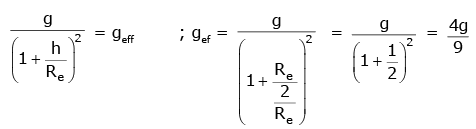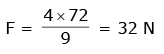Q.2. A body weighs 200 N on the surface of the earth. How much will it weigh half way down to the centre of the earth?    
A: 150 N
B: 200 N
C: 250 N
D: 100 N
Ans:
D
Solution: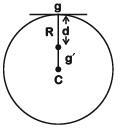Acceleration due to gravity at a depth d from surface of earth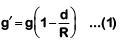Where g = acceleration due to gravity at earth's surface
Multiplying by mass 'm' on both sides of (1)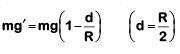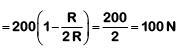Q.3. If the mass of the Sun were ten times smaller and the universal gravitational constant were ten time larger in magnitude, which of the following is not correct ?   
A: Raindrops will fall faster
B: Walking on the ground would become more difficult
C: Time period of a simple pendulum on the Earth would decrease
D: 'g' on the Earth will not change
Ans:
D
Solution: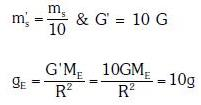Raindrops will fall faster, Walking on the ground would become more difficult. Time period of a simple pendulum on the earth would decrease.

Q.4. The acceleration due to gravity at a height 1 km above the earth is the same as at a depth d below the surface of earth. Then:-    
A: d = 1 km
B: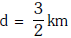C: d = 2 km
D: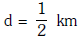Ans:
C
Solution: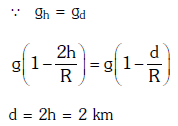Q.5. Two astronauts are floating in gravitational free space after having lost contact with their spaceship.The two will :-    
A: Move towards each other.
B: Move away from each other.
C: Will become stationary
D: Keep floating at the same distance between them.
Ans:
A
Solution: Astronauts move towards each other under mutual gravitational force.

Q.6. At what height from the surface of earth the gravitational potential and the value of g are -5.4 x 10-7J kg-2 and 6.0 ms-2 respectively ? Take the radius of earth as 6400 km.    
A: 2000 km
B: 2600 km
C: 1600 km
D: 1400 km
Ans:
B
Solution:
-GM/r = 5.4 x 10-7
-GM/r2 = 6.0
dividing both the equations, r = 9000 km.
so height from the surface = 9000 - 6400 = 2600 km

Q.7. The ratio of escape velocity at earth (ve) to the escape velocity at a planet (vp) whose radius and mean density are twice as that of earth is :    
A: 1:√2
B: 1:2
C: 1:2√2
D: 1:4
Ans:
C
Solution: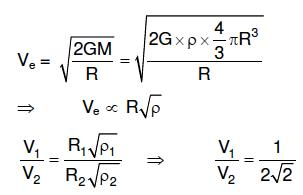Q.8. Kepler's third law states that square of period of revolution (T) of a planet around the sun, is proportional to third power of average distance r between sun and planet i.e. T2 = Kr3. here K is constant.
If the masses of sun and planet are M and m respectively then as per Newton?s law of gravitation force of attraction between them is
F= GMm/r2 , here G is gravitational constant. The relation between G and K is described as :     
A: K=1/G
B: GK4π2
C: GMK=4π2
D: K=G
Ans:
C
Solution: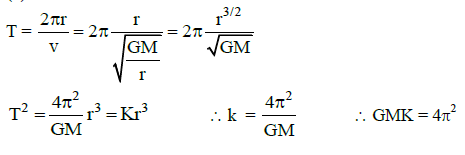Q.9. Two spherical bodies of mass M and 5 M and radii R and 2 R are released in free space with initial separation between their centres equal to 12 R. If they attract each other due to gravitational force only, then the distance covered by the smaller body before collision is :    
A: 1.5 R
B: 2.5 R
C: 4.5 R
D: 7.5 R
Ans:
D
Solution: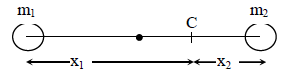Let centre of mass C at a distance x1 from m1 and x2 from m2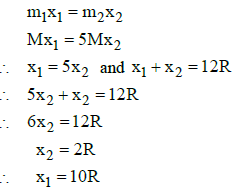Since the masses are moving under mutual attraction the position of centre of mass remains constant.
When the masses are in contact, let x'1 and x'2 be the distance of their centres from the centre of mass.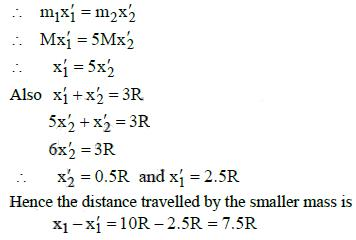Q.10. Dependence of intensity of gravitational field (E) of earth with distance (r) from centre of earth is correctly represented by:    
A: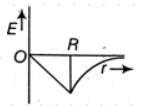B: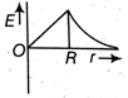C: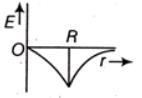D:Ans:
B

Q.11. A block hole is an object whose gravitational field is so strong that even light cannot escape from it. To what approximate radius would earth (mass = 5.98 × 1024 kg) have to be compressed to be a black hole ?   
A: 10−2 m
B: 100 m
C: 10-9 m
D: 10-6m
Ans:
A

Solution: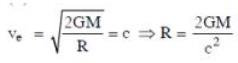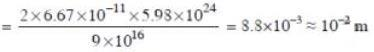Offer running on EduRev: Apply code STAYHOME200 to get INR 200 off on our premium plan EduRev Infinity!

14 docs|25 tests

,

,

,

,

,

,

,

,

,

,

,

,

,

,

,

,

,

,

,

,

,

;